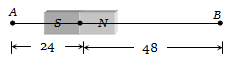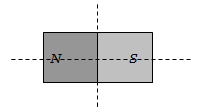A bar magnet of length 3 cm has points A and B along its axis at distances of 24 cm and 48 cm on the opposite sides. Ratio of magnetic fields at these points will be(a) 8                               (b)$\frac{1}{2\sqrt{2}}$

(c) 3                               (d) 4

Concept Questions :-

Earth's magnetism
High Yielding Test Series + Question Bank - NEET 2020

Difficulty Level:

A dip needle in a plane perpendicular to magnetic meridian will remain

(a) Vertical

(b) Horizontal

(c) In any direction

(d) At an angle of dip to the horizontal

Concept Questions :-

Earth's magnetism
High Yielding Test Series + Question Bank - NEET 2020

Difficulty Level:

If the angles of dip at two places are 30o and 45o respectively, then the ratio of horizontal  components of earth's magnetic field at the two places will be

(a) $\sqrt{3}:\sqrt{2}$                       (b) $1:\sqrt{2}$

(c) $1:\sqrt{3}$                          (d) 1:2

Concept Questions :-

Earth's magnetism
High Yielding Test Series + Question Bank - NEET 2020

Difficulty Level:

A line passing through places having zero value of magnetic dip is called

(a) Isoclinic line             (b) Agonic line

(c) Isogonic line             (d) Aclinic line

Concept Questions :-

Earth's magnetism

Difficulty Level:

The earth's magnetic field at a certain place has a horizontal component 0.3 Gauss and the total strength 0.5 Gauss. The angle of dip is

(a) ${\mathrm{tan}}^{-1}\left(\frac{3}{4}\right)$                      (b) ${\mathrm{sin}}^{-1}\left(\frac{3}{4}\right)$

(c) ${\mathrm{tan}}^{-1}\left(\frac{4}{3}\right)$                      (d) ${\mathrm{sin}}^{-1}\left(\frac{3}{5}\right)$

Concept Questions :-

Earth's magnetism
High Yielding Test Series + Question Bank - NEET 2020

Difficulty Level:

At a certain place, the horizontal component B0 and the vertical component V0 of the earth's magnetic field are equal in magnitude. The total intensity at the place will be

1. ${B}_{0}$                            2. ${B}_{0}^{2}$

3. $2{B}_{0}$                          4. $\sqrt{2}{B}_{0}$

Concept Questions :-

Earth's magnetism
High Yielding Test Series + Question Bank - NEET 2020

Difficulty Level:

Two bar magnets with magnetic moments 2 M and M are fastened together at right angles to each other at their centres to form a crossed system, which can rotate freely about a vertical axis through the centre. The crossed system sets in earth’s magnetic field with magnet having magnetic moment 2M making an angle $\theta$ with the magnetic meridian such that

(a) $\theta ={\mathrm{tan}}^{-1}\left(\frac{1}{\sqrt{3}}\right)$            (b) $\theta ={\mathrm{tan}}^{-1}\left(\sqrt{3}\right)$

(c) $\theta ={\mathrm{tan}}^{-1}\left(\frac{1}{2}\right)$               (d) $\theta ={\mathrm{tan}}^{-1}\left(\frac{3}{4}\right)$

Concept Questions :-

Earth's magnetism
High Yielding Test Series + Question Bank - NEET 2020

Difficulty Level:

The angle of dip at a certain place is 30o. If the horizontal component of the earth’s magnetic field is H, the intensity of the total magnetic field is

1. $\frac{H}{2}$                            2. $\frac{2H}{\sqrt{3}}$

3. $H\sqrt{2}$                         4. $H\sqrt{3}$

Concept Questions :-

Earth's magnetism
High Yielding Test Series + Question Bank - NEET 2020

Difficulty Level:

The time period of oscillation of a freely suspended bar magnet with usual notations is given by

1. $T=2\mathrm{\pi }\sqrt{\frac{\mathrm{I}}{{\mathrm{MB}}_{\mathrm{H}}}}$

2.  $T=2\mathrm{\pi }\sqrt{\frac{M{B}_{H}}{I}}$

3. $T=2\sqrt{\frac{\mathrm{I}}{{\mathrm{MB}}_{\mathrm{H}}}}$

4. $T=2\mathrm{\pi }\sqrt{\frac{{B}_{H}}{MI}}$

Concept Questions :-

Bar magnet
High Yielding Test Series + Question Bank - NEET 2020

Difficulty Level:

Time period for a magnet is T. If it is divided in four equal parts along its axis and perpendicular to its axis as shown then time period for each part will be1. 4T

2. T/4

3. T/2

4. T

Concept Questions :-

Bar magnet